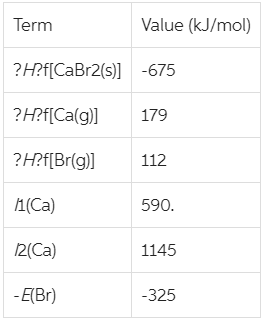# Problem: Given the following thermodynamic data, calculate the lattice energy of CaBr2(s).Express your answer in kilojoules per mole using four significant figures.

###### FREE Expert Solution

Lattice energy   → required to combine two gaseous ions into a solid ionic compound:

100% (479 ratings)###### Problem Details

Given the following thermodynamic data, calculate the lattice energy of CaBr2(s).